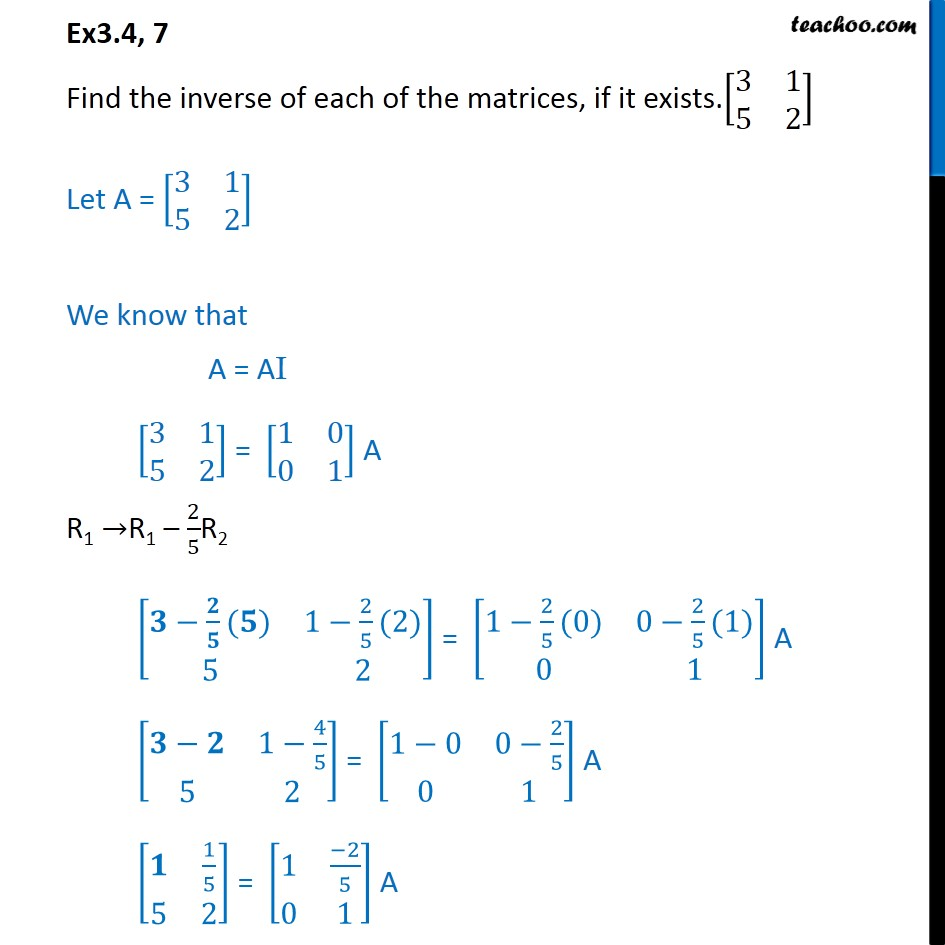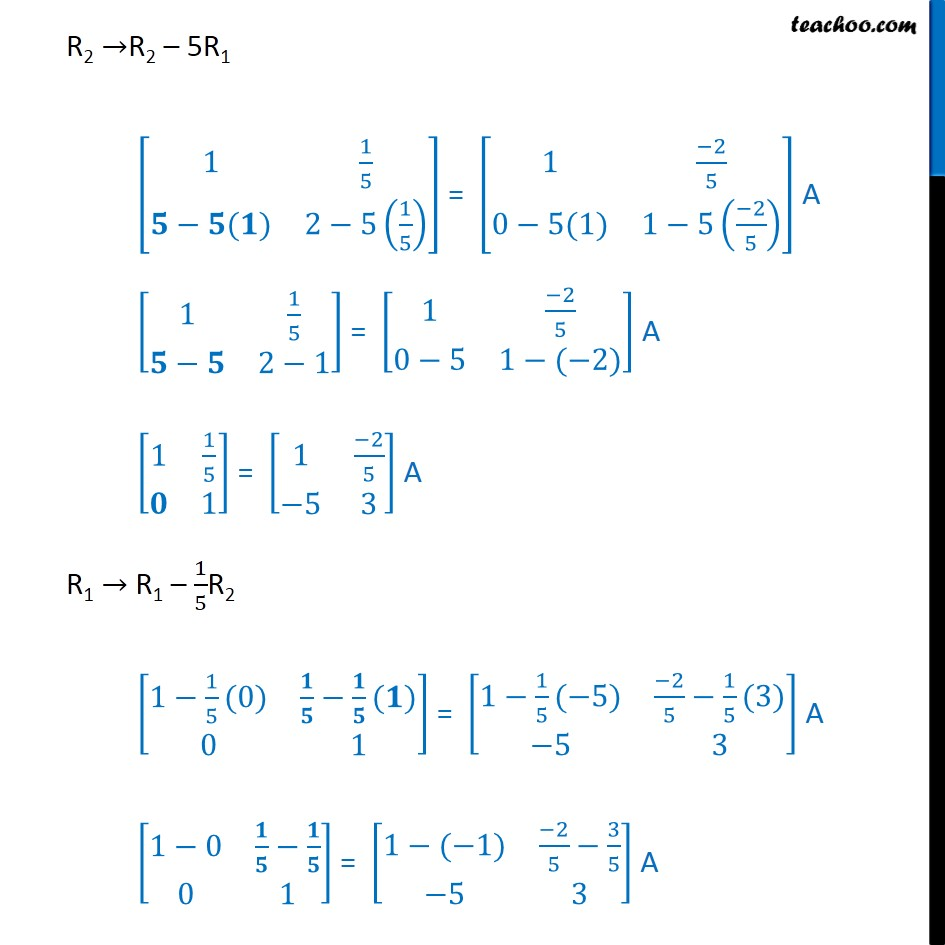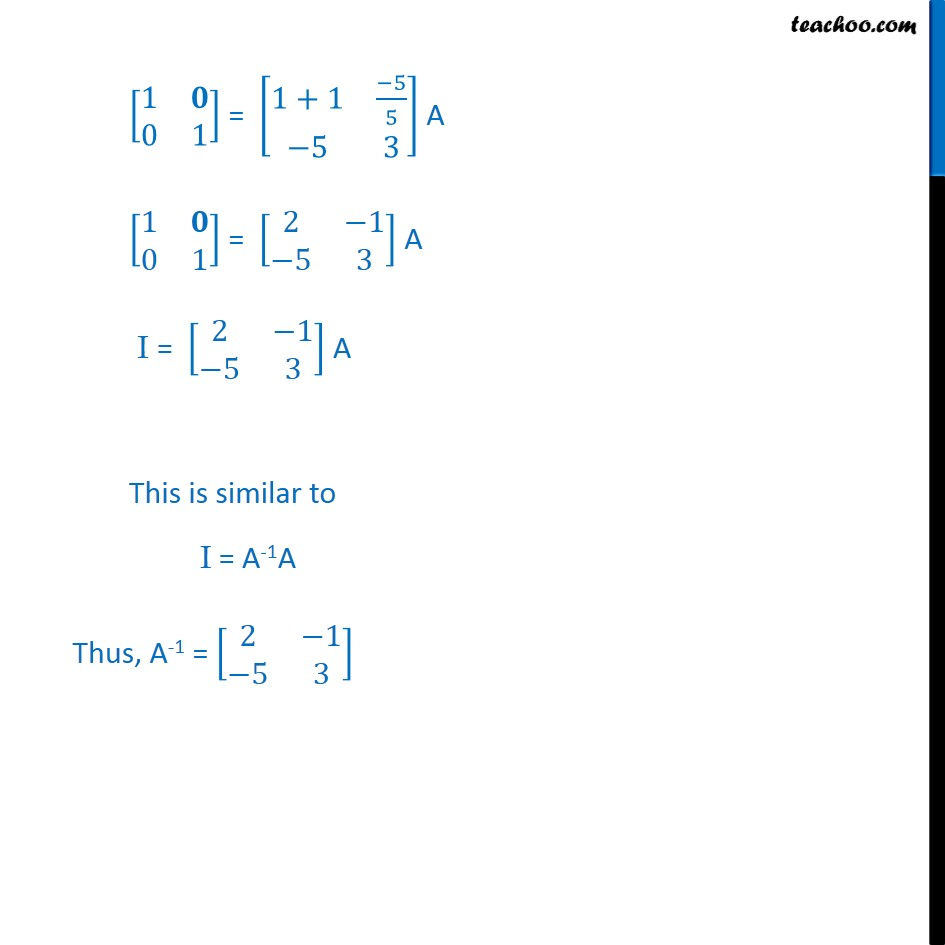Ex 3.4

Chapter 3 Class 12 Matrices (Term 1)
Serial order wise### Transcript

Ex3.4, 7 Find the inverse of each of the matrices, if it exists.[■8(3&1@5&2)] Let A = [■8(3&1@5&2)] We know that A = AI [■8(3&1@5&2)] = [■8(1&0@0&1)] A R1 →R1 – 2/5R2 [■8(𝟑−𝟐/𝟓(𝟓)&1−2/5(2)@5&2)] = [■8(1−2/5(0)&0−2/5(1)@0&1)] A [■8(𝟑−𝟐&1−4/5@5&2)] = [■8(1−0&0−2/5@0&1)] A [■8(𝟏&1/5@5&2)] = [■8(1&(−2)/5@0&1)] A R2 →R2 – 5R1 [■8(1&1/5@𝟓−𝟓(𝟏)&2−5(1/5) )] = [■8(1&(−2)/5@0−5(1)&1−5((−2)/5) )] A [■8(1&1/5@𝟓−𝟓&2−1)] = [■8(1&(−2)/5@0−5&1−(−2))] A [■8(1&1/5@𝟎&1)] = [■8(1&(−2)/5@−5&3)] A R1 → R1 – 1/5R2 [■8(1−1/5(0)&𝟏/𝟓−𝟏/𝟓(𝟏)@0&1)] = [■8(1−1/5(−5)&(−2)/5−1/5(3)@−5&3)] A [■8(1−0&𝟏/𝟓−𝟏/𝟓@0&1)] = [■8(1−(−1)&(−2)/5−3/5@−5&3)] A [■8(1&𝟎@0&1)] = [■8(1+1&(−5)/5@−5&3)] A [■8(1&𝟎@0&1)] = [■8(2&−1@−5&3)] A I = [■8(2&−1@−5&3)] A This is similar to I = A-1A Thus, A-1 = [■8(2&−1@−5&3)]